# The Law of Cosines

### Article objectives

• To learn about the Law of Cosines for triangles.
• For a general triangle, which may or may not have a right angle, we will again need three pieces of information. The four cases are:

Case 1: One side and two angles
Case 2: Two sides and one opposite angle
Case 3: Two sides and the angle between them
Case 4: Three sides

Let's discuss Case 3: how to solve a triangle with two sides and the angle between them. First, let us see what happens when we try to use the Law of Sines for this case.

Example 1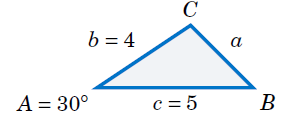Case 3: Two sides and the angle between them. Solve the triangle ∆ABC given A = 30º, b = 4, and c = 5.

Solution: Using the Law of Sines, we have $$\frac{a}{ sin 30^\circ} = \frac{4}{ sin B} = \frac{5}{ sin C} ,$$

where each of the equations has two unknown parts, making the problem impossible to solve. For example, to solve for a we could use the equation $$\frac{4}{sin B}$$ = $$\frac{5}{sin C}$$ to solve for sin B in terms of sin C and substitute that into the equation $$\frac{a}{sin 30º}$$ = $$\frac{4}{ sin B}$$ . But that would just result in the equation $$\frac{a}{sin 30º}$$ = $$\frac{5}{sin C}$$ , which we already knew and which still has two unknowns! Thus, this problem can not be solved using the Law of Sines.

To solve the triangle in the above example, we can use the Law of Cosines:

Theorem 1: Law of Cosines: If a triangle has sides of lengths a, b, and c opposite the angles A, B, and C*, respectively, then

$$a^2 = b^2 + c^2 − 2bc\;cos A , (1)$$ $$b^2 = c^2 + a^2 − 2ca\;cos B , (2)$$ $$c^2 = a^2 + b^2 − 2ab\;cos C . (3)$$

To prove the Law of Cosines, let ∆ABC be an oblique triangle. Then ∆ABC can be acute, as in Figure 2, or it can be obtuse, as in Figure 3. In each case, draw the altitude from the vertex at C to the side $$\overline{AB}$$. In Figure 2 the altitude divides $$\overline{AB}$$ into two line segments with lengths x and cx, while in Figure 3 the altitude extends the side $$\overline{AB}$$ by a distance x. Let h be the height of the altitude.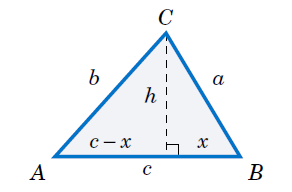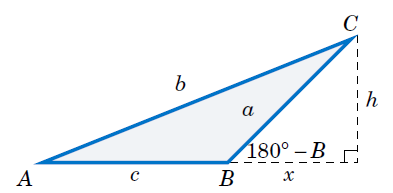For each triangle in Figures in 2 & 3, we see by the Pythagorean Theorem that

$$h^2 = a^2 − x^2 (4)$$

and likewise for the acute triangle in Figure 2 we see that

$$b^2 = h^2 + (c−x)^2 . (5)$$

Thus, substituting the expression for $$h^{2}$$ in equation (4) into equation (5) gives

$$b^2 = a^2 − x^2 + (c−x)^2$$ $$= a^2 − x^2 + c^2 − 2cx + x^2$$ $$= a^2 + c^2 − 2cx$$

But we see from Figure 2 that x = a cos B, so

$$b^2 = a^2 + c^2 − 2ca\;cos B . (6)$$

And for the obtuse triangle in Figure 3 we see that

$$b^2 = h^2 + (c+x)^2 . (7)$$

Thus, substituting the expression for $$h^{2}$$ in equation (4) into equation (7) gives

$$b^2 = a^2 − x^2 + (c+x)^2$$ $$= a^2 − x^2 + c^2 + 2cx + x^2$$ $$= a^2 + c^2 + 2cx .$$

But we see from Figure 2 that x = a cos (180º−B), and we know that cos (180º −B) = −cos B. Thus, x = −a cos B and so

$$b^2 = a^2 + c^2 − 2ca\;cos B . (8)$$

So for both acute and obtuse triangles we have proved formula (2) in the Law of Cosines. Notice that the proof was for B acute and obtuse. By similar arguments for A and C we get the other two formulas. Note that we did not prove the Law of Cosines for right triangles, since it turns out that all three formulas reduce to the Pythagorean Theorem for that case. The Law of Cosines can be viewed as a generalization of the Pythagorean Theorem. Also, notice that it suffices to remember just one of the three formulas (2-4), since the other two can be obtained by “cycling” through the letters a, b, and c. That is, replace a by b, replace b by c, and replace c by a (likewise for the capital letters). One cycle will give you the second formula, and another cycle will give you the third.

The angle between two sides of a triangle is often called the included angle. Notice in the Law of Cosines that if two sides and their included angle are known (e.g. b, c, and A), then we have a formula for the square of the third side. We will now solve the triangle from Example 1.

Example 2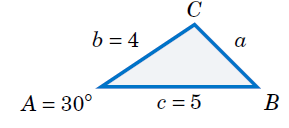Case 3: Two sides and the angle between them. Solve the triangle ∆ABC given A = 30º, b = 4, and c = 5.

Solution: We will use the Law of Cosines to find a, use it again to find B, then use C = 180º − AB. First, we have

$$a^2 = b^2 + c^2 − 2bc\;cos A$$ $$= 4^2 + 5^2 − 2(4)(5) cos 30^\circ = 6.36 ⇒ \boxed{a = 2.52} .$$

Now we use the formula for $$b^{2}$$ to find B:

$$b^2 = c^2 + a^2 − 2ca cos B ⇒ cos B = \frac{c^2 + a^2 − b^2}{ 2ca}$$ $$⇒ cos B = \frac{5^2 + (2.52)^2 − 4^2}{ 2(5)(2.52)} = 0.6091$$ $$⇒ \boxed{B = 52.5^\circ}$$

Thus, C = 180º − AB = 180º −30º −52.5º ⇒ C = 97.5º .

Notice in Example 2 that there was only one solution. For Case 3 this will always be true: when given two sides and their included angle, the triangle will have exactly one solution. The reason is simple: when joining two line segments at a common vertex to form an angle, there is exactly one way to connect their free endpoints with a third line segment, regardless of the size of the angle.

You may be wondering why we used the Law of Cosines a second time in Example 2, to find the angle B. Why not use the Law of Sines, which has a simpler formula? The reason is that using the cosine function eliminates any ambiguity: if the cosine is positive then the angle is acute, and if the cosine is negative then the angle is obtuse. This is in contrast to using the sine function; both an acute angle and its obtuse supplement have the same positive sine.

To see this, suppose that we had used the Law of Sines to find B in Example 2:

$$sin B = \frac{b sin A} {a} = \frac{4 sin 30^\circ} {2.52} = 0.7937 ⇒ B = 52.5^\circ or 127.5^\circ$$

How would we know which answer is correct? We could not immediately rule out B = 127.5º as too large, since it would make A +B = 157.5º < 180º and so C = 22.5º, which seems like it could be a valid solution. However, this solution is impossible. Why? Because the largest side in the triangle is c = 5, which means that C has to be the largest angle. But C = 22.5º would not be the largest angle in this solution, and hence we have a contradiction.

It remains to solve a triangle in Case 4, i.e. given three sides. We will now see how to use the Law of Cosines for that case.

Example 3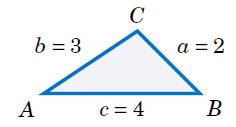Case 4: Three sides. Solve the triangle ∆ABC given a = 2, b = 3, and c = 4.

Solution: We will use the Law of Cosines to find B and C, then use A = 180º −BC. First, we use the formula for $$b^{2}$$ to find B: $$b^2 = c^2 + a^2 − 2ca cos B ⇒ cos B = \frac{c^2 + a^2 − b^2}{2ca}$$ $$⇒ cos B = \frac{4^2 + 2^2 − 3^2}{ 2(4)(2)} = 0.6875$$ $$\boxed{⇒ B = 46.6^\circ}$$

Now we use the formula for $$c^{2}$$ to find C: $$c^2 = a^2 + b^2 − 2ab\;cos C ⇒ cos C = \frac{a^2 + b^2 − c^2}{ 2ab}$$ $$⇒ cos C = \frac{2^2 + 3^2 − 4^2}{ 2(2)(3)} = −0.25$$ $$\boxed{⇒ C = 104.5^\circ}$$

Thus, A = 180º −BC = 180º −46.6º −104.5º⇒ $$\boxed{A = 28.9º}$$ .

It may seem that there is always a solution in Case 4 (given all three sides), but that is not true, as the following example shows.

Example 4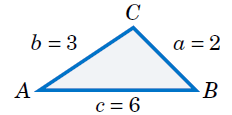Case 4: Three sides. Solve the triangle ∆ABC given a = 2, b = 3, and c = 6.

Solution: If we blindly try to use the Law of Cosines to find A, we get

$$a^2 = b^2 + c^2 − 2bc cos A ⇒ cos A = \frac{b^2 + c^2 − a^2}{2bc} = \frac{3^2 + 6^2 − 2^2}{2(3)(6)} = 1.139 ,$$

which is impossible since |cos A| ≤ 1. Thus, there is $$\boxed{\text{no solution}}$$ .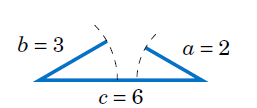We could have saved ourselves some effort by recognizing that the length of one of the sides (c = 6) is greater than the sums of the lengths of the remaining sides (a = 2 and b = 3), which (as the picture on the right shows) is impossible in a triangle.

The Law of Cosines can also be used to solve triangles in Case 2 (two sides and one opposite angle), though it is less commonly used for that purpose than the Law of Sines. The following example gives an idea of how to do this.

Example 5

Case 2: Two sides and one opposite angle. Solve the triangle ∆ABC given a = 18, A = 25º, and b = 30.

Solution: To solve this using the Law of Cosines, first find c by using the formula for a2:

$$a^2 = b^2 + c^2 − 2bc\;cos A ⇒ 18^2 = 30^2 + c^2 − 2(30)c cos 25^\circ$$ $$⇒ c^2 − 54.38 c + 576 = 0 ,$$

which is a quadratic equation in c, so we know that it can have either zero, one, or two real roots (corresponding to the number of solutions in Case 2). By the quadratic formula, we have

$$c =\frac{\sqrt{54.38 ±(54.38)^2 − 4(1)(576)}} {2(1)} = 40 or 14.4 .$$

Note that these are the same values for c that we found before. For c = 40 we get

$$cos B = \frac{c^2 + a^2 − b^2}{ 2ca} = \frac{40^2 + 18^2 − 30^2}{ 2(40)(18)} = 0.7111 ⇒ B = 44.7^\circ ⇒ C = 110.3^\circ ,$$

which is close to what we found before (the small difference being due to different rounding). The other solution set can be obtained similarly.

Like the Law of Sines, the Law of Cosines can be used to prove some geometric facts, as in the following example.

Example 6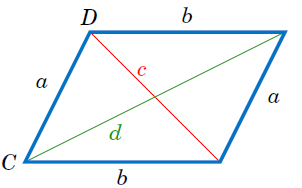Use the Law of Cosines to prove that the sum of the squares of the diagonals of any parallelogram equals the sum of the squares of the sides.

Solution: Let a and b be the lengths of the sides, and let the diagonals opposite the angles C and D have lengths c and d, respectively, as in Figure 8. Then we need to show that

$$c^2 + d^2 = a^2 + b^2 + a^2 + b^2 = 2(a^2 + b^2) .$$

By the Law of Cosines, we know that $$c^2 = a^2 + b^2 − 2ab cos C , and$$ $$d^2 = a^2 + b^2 − 2ab cos D .$$

By properties of parallelograms, we know that D = 180º −C, so $$d^2 = a^2 + b^2 − 2ab cos (180^\circ −C)$$ $$= a^2 + b^2 + 2ab cos C ,$$

since cos (180º −C) = −cos C. Thus,

$$c^2 + d^2 = a^2 + b^2 − 2ab cos C + a^2 + b^2 + 2ab\;cos C$$ $$= 2(a^2 + b^2) .$$